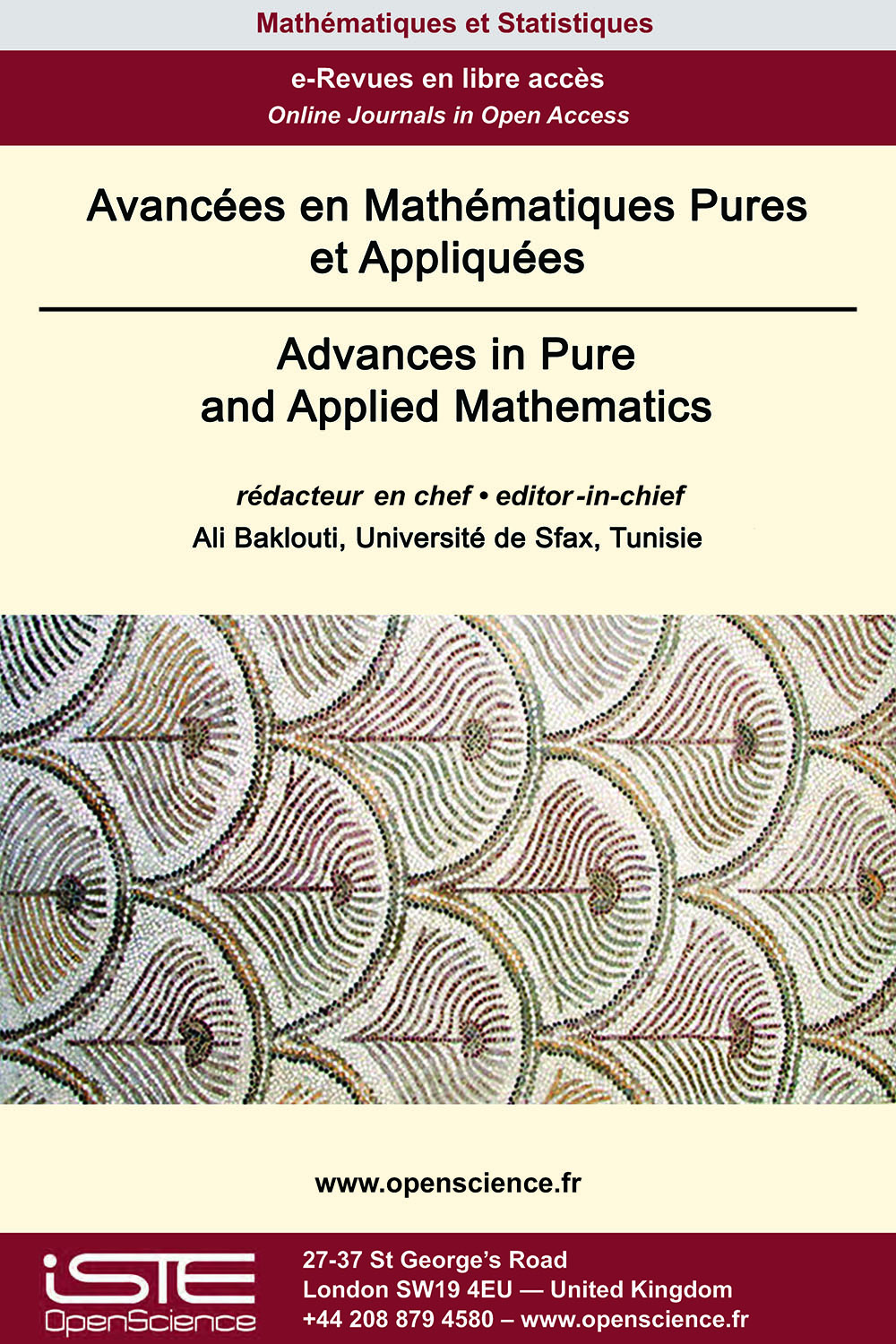# Vol 11 - Numéro 2 (Septembre 2020)

## Articles parus

Classes héréditaires des structures binaires ordonnées

Balogh, Bollobás and Morris (2006) have described a threshold phenomenon in the behavior of the profile of hereditary classes of ordered graphs. In this paper, we give an other look at their result based on the notion of monomorphic decomposition of a relational structure introduced in . We prove that the class 𝔖 of ordered binary structures which do not have a finite monomorphic decomposition has a finite basis (a subset 𝔄 such that every member of 𝔖 embeds some member of 𝔄). In the case of ordered reflexive directed graphs, the basis has 1242 members and the profile of their ages grows at least as the Fibonacci function. From this result, we deduce that the following dichotomy property holds for every hereditary class ℭ of finite ordered binary structures of a given finite type. Either there is an integer ℓ such that every member of ℭ has a monomorphic decomposition into at most ℓ blocks and in this case the profile of ℭ is bounded by a polynomial of degree ℓ − 1 (and in fact is a polynomial), or ℭ contains the age of a structure which does not have a finite monomorphic decomposition, in which case the profile of ℭ is bounded below by the Fibonacci function.

Sur certains systèmes elliptiques non locaux à paramètres multiples

In this paper, using sub-supersolution argument, we prove some existence results on positive solution for a class of nonlocal elliptic systems with multiple parameters in bounded domains.

Sur les valeurs propres de Sketlov pour certains *-forme domaines

In this article, we consider Steklov eigenvalue problem on star-shaped bounded domain Ω in hypersurface of revolution and paraboloid, P = {(x, y, z) ∈ ℝ3 : z = x2 + y2}. A sharp lower bound is derived for all Steklov eigenvalues of Ω in terms of the Steklov eigenvalues of the largest geodesic ball contained in Ω with the same center as Ω. This work is a generalization of a result given by Kuttler and Sigillito (SIAM Rev 10:368 − 370, 1968) on a star-shaped bounded domain in ℝ2.

Solutions globales pour un système d’équations de chaleur semi-linéaires et système correspondant d’équations d’ondes amorties

We consider the Cauchy problem for a strongly coupled semi-linear heat equations with some kind of nonlinearity in multi-dimensional space ℝN. We see under some conditions on the exponents and on the dimension N, that the existence and uniqueness of time-global solutions for small data and their asymptotic behaviors are obtained. This observation will be applied to the corresponding system of the damped wave equations in low dimensional space.### Autres numéros :

2020

Volume 20- 11

Numéro 1 (Mai 2020)
Numéro 2 (Septembre 2020)

2021

Volume 21- 12

Numéro 1 (Janvier 2021)
Numéro 2 (Mai 2021)
Numéro spécial : AUS-ICMS 2020
Numéro 3 (Septembre 2021)

2022

Volume 22- 13

À paraître

Numéro 1 (Janvier 2022)
Numéro 2 (Mars 2022)
Numéro 3 (Juin 2022)
Numéro 4 (Septembre 2022)

2023

Volume 23- 14

Numéro 1 (Janvier 2023)
Numéro 2 (Spécial CSMT 2022)